NEET  >  DC Pandey Solutions: Sound Waves - 1

# DC Pandey Solutions: Sound Waves - 1 - Notes | Study Physics Class 11 - NEET

 Table of contentsIntroductory Exercise 16.1Introductory Exercise 16.2Introductory Exercise 16.31 Crore+ students have signed up on EduRev. Have you?

Introductory Exercise 16.1

Q.1: Calculate the bulk modulus of air from the following data for a sound wave of wavelength 35 cm travelling in air. The pressure at a point varies between (105 ± 14) Pa and the particles of the air vibrate in SHM of amplitude 5.5 × 10-6 m.
Sol: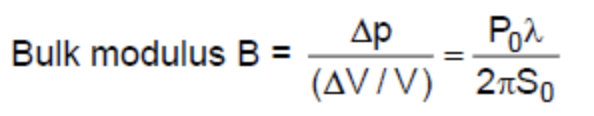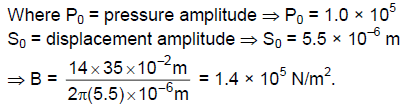Q.2: Find the minimum and maximum wavelengths of sound in water that is in the audible range for an average human ear. Speed of sound in water is 1450 m/s.
Sol:
(a) For minimum wavelength n = 20 KHz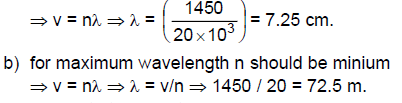Q.3: A typical loud sound wave with a frequency of 1 kHz has a pressure amplitude of about 10 Pa (a) At t = 0, the pressure is a maximum at some point x1. What is the displacement at that point at t = 0?
(b) What is the maximum value of the displacement at any time and place ? Take the density of air to be 1.29 kg/m3 and speed of sound in air is 340 m/s.
Sol:
(a) Displacement is zero when pressure is maximum.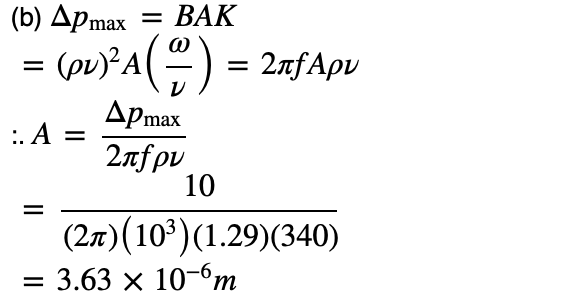Q.4:  The pressure variation in a sound wave in air is given by
ΔP = 12 sin(8.18x - 2700t +π/4) N/m2
Find the displacement amplitude. Density of air = 1.29 kg/m3.
Sol:
In the above problem, we have found that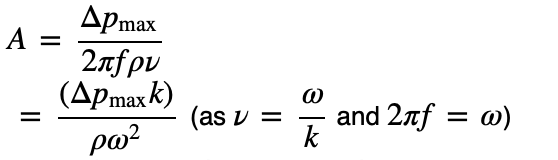Now substituting the value, we have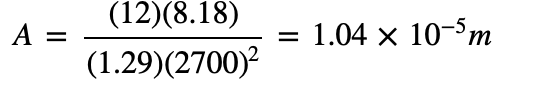Introductory Exercise 16.2

Q.1: Calculate the temperature at which the velocity of sound in air is double its velocity at 0°C.
Sol:
Speed of velocity in air is, v ∝ √T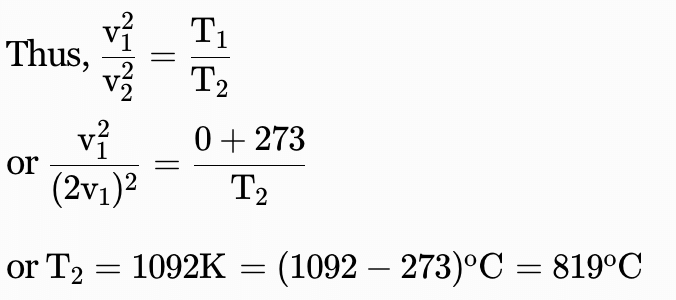Q.2: Calculate the difference in the speeds of sound in air at -3°C, 60 cm pressure of mercury and 30°C, 75 cm pressure of mercury. The speed of sound in air at 0°C is 332 m/s.
Sol:
v ∝ √T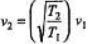= 330.17 m/s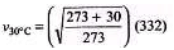= 349.77 m/s
The difference in these two speeds is approximately 20 m/s.

Q.3: In a liquid wit h density 900 kg/m3, longitudinal waves with frequency 250 Hz are found to have wavelength 8.0 m. Calculate the bulk modulus of the liquid.
Sol: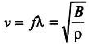∴ B = ρ (fλ)2
= (900) (250 × 8)2 = 3.6 × 109 N/m2

Q.4: Calculate the speed of sound in oxygen at 273 K.
Sol: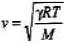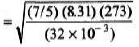= 315 m/s

Introductory Exercise 16.3

Q.1: A sound wave in air has a frequency of 300 Hz and a displacement amplitude of 6.0 × 10 -3 mm. For this sound wave calculate the
(a) pressure amplitude
(b) intensity
(c) sound intensity level (in dB)
Speed of sound = 344 m/s and density of air = 1.2 kg/m3.

Sol: (a)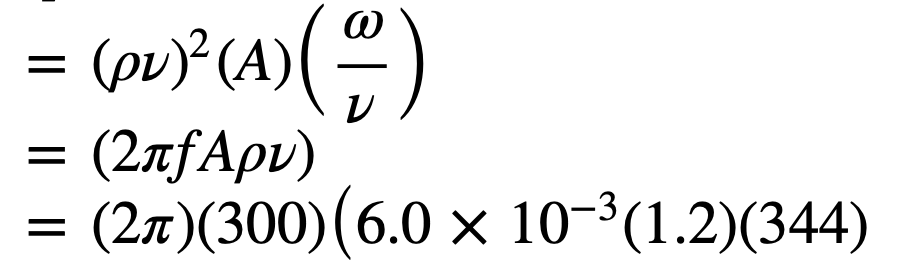= 4.67 Pa

(b)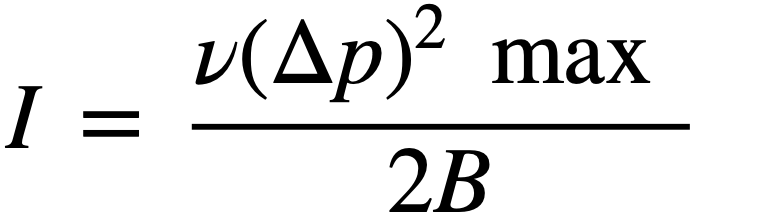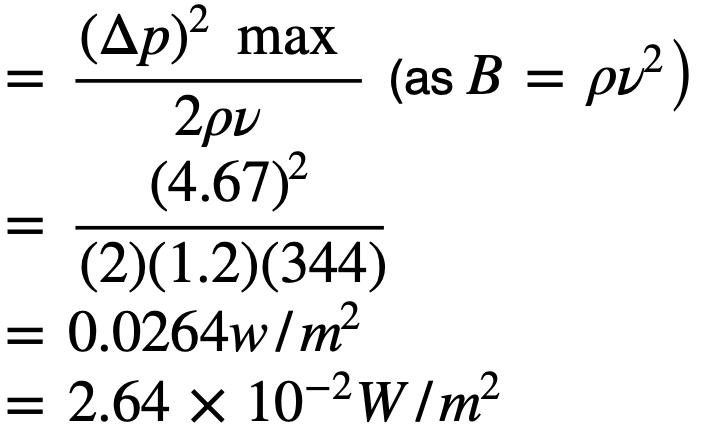(c)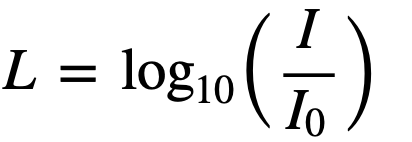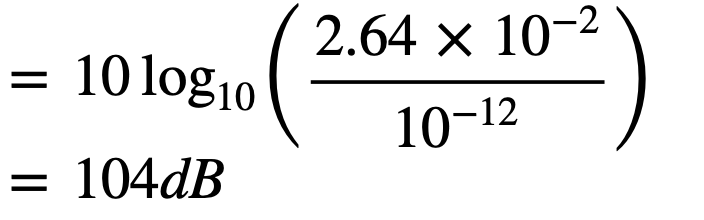Q.2: Most people interpret a 9.0 dB increase in sound intensity level as a doubling in loudness. By what factor must the sound intensity be increased to double the loudness?Sol: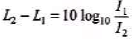Given L2 - L1 =9 dB
Solving the equation we get,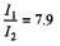Q.3: A baby's mouth is 30 cm from her father's ear and 3.0 m from her mother's ear. What is the, difference between the sound intensity levels heard by the father and by the mother.
Sol: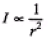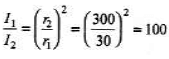Now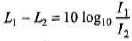Substituting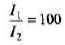We get, Lx1 - L2 - 20 dB

Q.4: The faintest sound that can be heard has a pressure amplitude of about 2 × 10-5 N/m2 and the loudest that can be heard without pain has a pressure amplitude of about 28 N/m2. Determine in each case
(a) the intensity of the sound both in W/mand in dB and
(b) the amplitude of the oscillations if the frequency is 500 Hz. Assume an air density of 1.29 kg/mand a velocity of sound is 345 m/s.
Sol: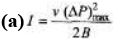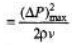For finest sound,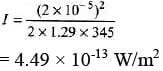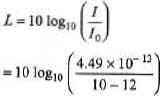= - 3.48 dB
Same formulae can be applied for loudest sound.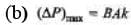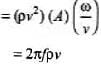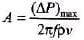For finest sound,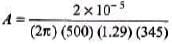= 1.43 × 10-11 m

The document DC Pandey Solutions: Sound Waves - 1 - Notes | Study Physics Class 11 - NEET is a part of the NEET Course Physics Class 11.
All you need of NEET at this link: NEET

## Physics Class 11

127 videos|464 docs|210 tests

## Physics Class 11

127 videos|464 docs|210 tests

### How to Prepare for NEET

Read our guide to prepare for NEET which is created by Toppers & the best Teachers

Track your progress, build streaks, highlight & save important lessons and more!

,

,

,

,

,

,

,

,

,

,

,

,

,

,

,

,

,

,

,

,

,

;